# Isosceles

A triangle in which two sides have the same length.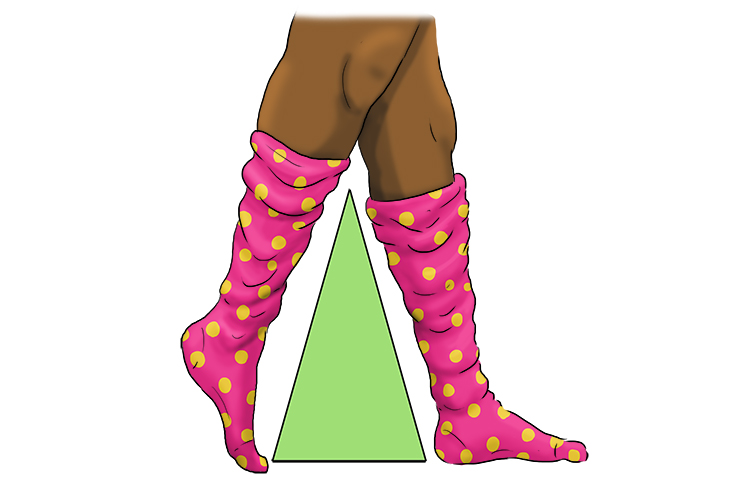"I so love these (isosceles) socks" can be used to remind you that two sides or two lengths of socks are equal.

Examples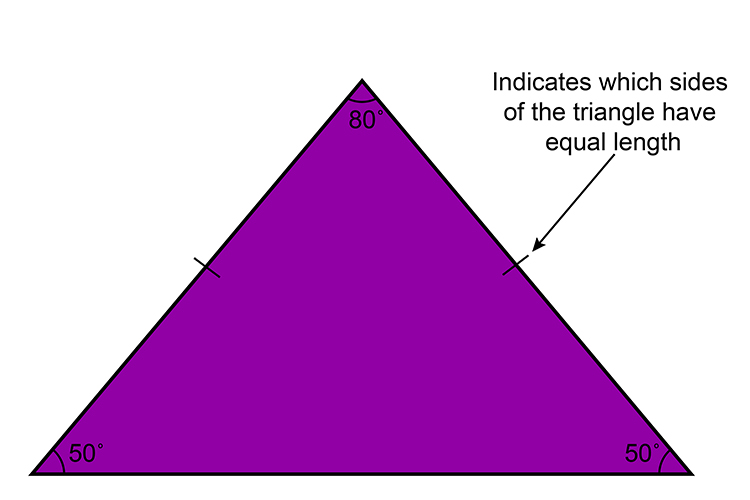An isosceles triangle with two sides same length giving two angles at 50° and one at 80°.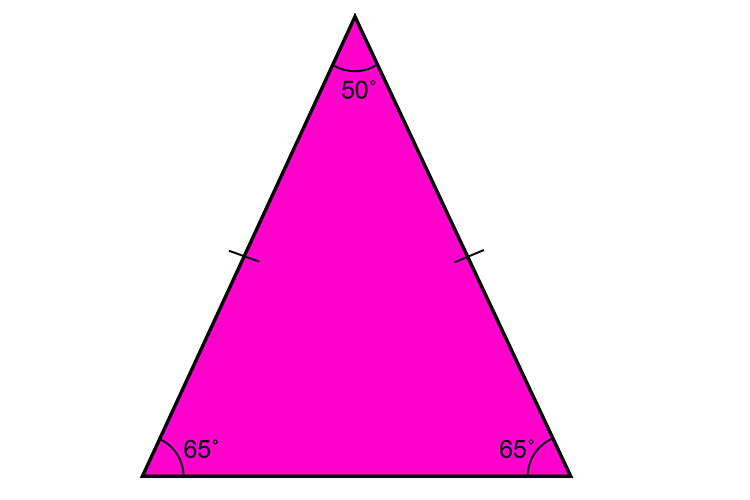An isosceles triangle with two sides same length giving two angles at 65° and one at 50°.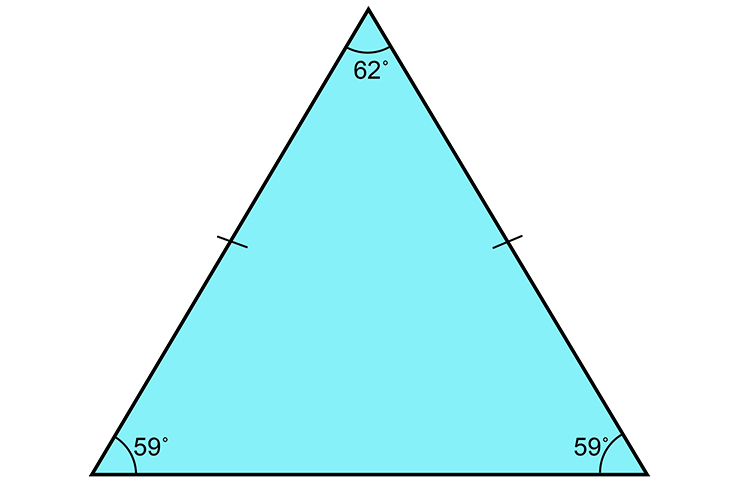An isosceles triangle with two sides same length giving two angles at 59° and one at 62°.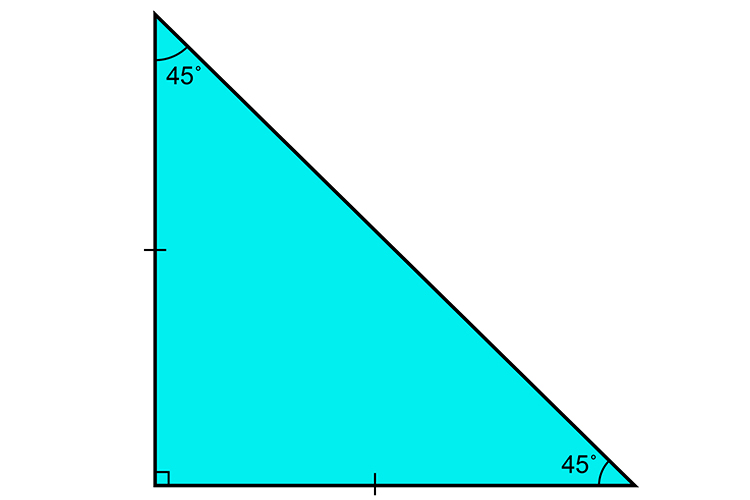A right angled triangle can be an isosceles as long as two of the sides are the same length.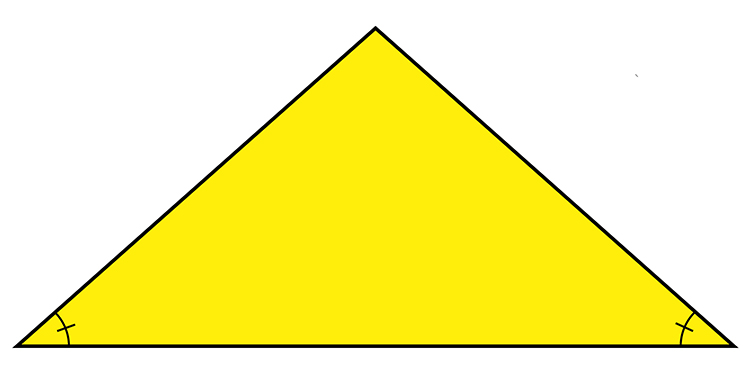A triangle with two angles the same will be an isosceles triangle.

NOTE:

Isosceles triangles have two internal angles that are the same and one which is different.

Remember to spell isosceles with an 'SOS' in the word.Even when stranded on an island, she wore the socks and sent out an SOS for another pair.